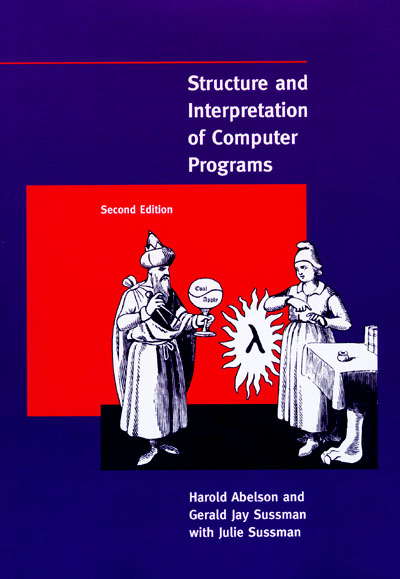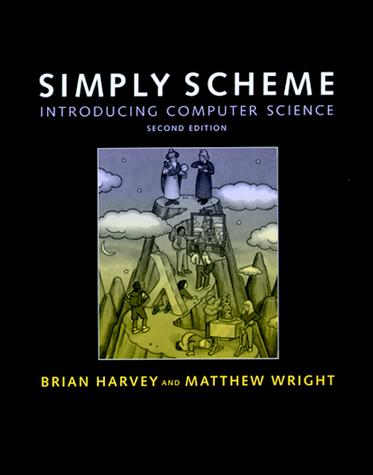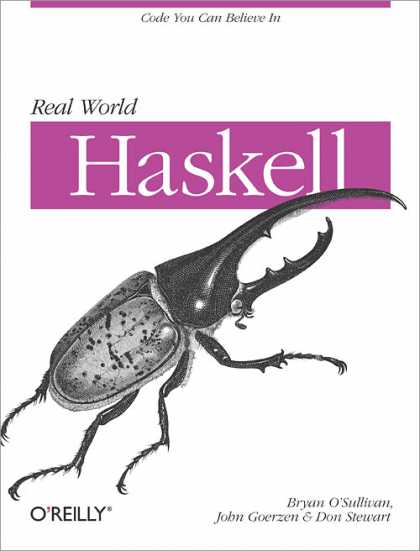## 老赵书托（2）：计算机程序的构造与解释

2009-07-15 00:11  Jeffrey Zhao  阅读(...)  评论(... 编辑 收藏

# 抽象• 您是否可以把一个多级的系统分类，理解为一颗树，然后用树或图的方式来处理它？
• 如果让您解八皇后问题，或者走一个简单的迷宫，基本上不太会难倒你，但是您可以把自己的思路使用程序表现出来吗？
• 领域驱动设计的一个重要部分，便是将真实世界中的“领域”提炼成模型，再使用计算机语言实现出来。

Thus, when we describe a language, we should pay particular attention to the means that the language provides for combining simple ideas to form more complex ideas. Every powerful language has three mechanisms for accomplishing this:

• primitive expressions, which represent the simplest entities the language is concerned with,
• means of combination, by which compound elements are built from simpler ones, and
• means of abstraction, by which compound elements can be named and manipulated as units.

# 函数式编程

static int Sum(int a, int b)
{
int sum = 0;
for (int i = a; i <= b; i++) sum += i;
return sum;
}

static int Sum(Func<int, int> f, int a, int b)
{
int sum = 0;
for (int i = a; i <= b; i++) sum += f(i);
return sum;
}

int i = Sum(x => x * x, 1, 3); // 14
int j = Sum(x => Math.Abs(x), -3, 3); // 12

static Func<int, int, int> SumCurry(Func<int, int> f)
{
return (a, b) => Sum(f, a, b);
}

var sumOfSquare = SumCurry(x => x * x); // int i = sumOfSquare(1, 3);
var sumOfCube = SumCurry(x => x * x * x); // int j = sumOfCube(1, 3);

# 补充建议# 相关文章

public static Func<T2, T3, TResult> Currying<T1, T2, T3, TResult>(
this Func<T1, T2, T3, TResult> f, T1 t1)
{
return (t2, t3) => f(t1, t2, t3);
}